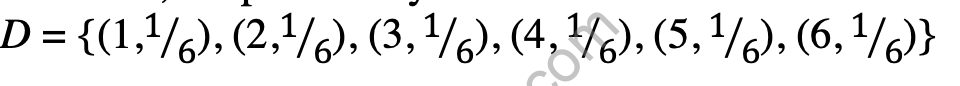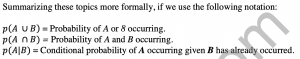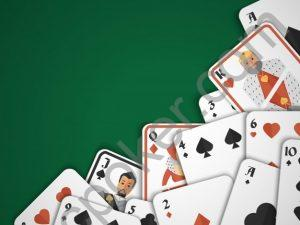# Probability DistributionsThough single event probabilities are important, it is often the case that they are inadequate to fully analyze a situation. Instead, it is frequently important to consider many different probabilities at the same time. We can characterize the possible outcomes and their probabilities from an event as a probability distribution.

Consider a fair coin flip. The coin flip has just two possible outcomes – each outcome is mutually exclusive and has a probability of 1/2.

We can create a probability distribution for the coin flip by taking each outcome and pairing it with its probability. So we have two pairs: (heads, 1/2 ) and (tails, 1/2 ).

If C is the probability distribution of the result of a coin flip, then we can write this as: C = {(heads, 1/2 ), (tails, 1/2 )}

Likewise, the probability distribution of the result of a fair six-sided die roll is:

We can construct a discrete probability distribution for any event by enumerating an exhaustive and mutually exclusive list of possible outcomes and pairing these outcomes with their corresponding probabilities.

We can therefore create different probability distributions from the same physical event. From our die roll we could also create a second probability distribution, this one the distribution of the odd-or-evenness of the roll:

In poker, we are almost always very concerned with the contents of our opponents’ hands. But it is seldom possible to narrow down our estimate of these contents to a single pair of cards. Instead, we use a probability distribution to represent the hands he could possibly hold and the corresponding probabilities that he holds them. At the beginning of the hand, before anyone has looked at their cards, each player’s probability distribution of hands is identical. As the hand progresses, however, we can incorporate new information we gain through the play of the hand, the cards in our own hand, the cards on the board, and so on, to continually refine the probability estimates we have for each possible hand.

Sometimes we can associate a numerical value with each element of a probability distribution. For example suppose that a friend offers to flip a fair coin with you. The winner will collect \$10 from the loser. Now the results of the coin flip follow the probability distribution we identified earlier:

Since we know the coin is fair, it doesn’t matter who calls the coin or what they call, so we can identify a second probability distribution that is the result of the bet:

We can then go further, and associate a numerical value with each result. If we win the flip, our friend pays us \$10. If we lose the flip, then we pay him \$10. So we have the following:

When a probability distribution has numerical values associated with each of the possible outcomes, we can find the expected value (EV) of that distribution, which is the value of each outcome multiplied by its probability, all summed together. Throughout the text, we will use the notation <X> to denote “the expected value of X.” For this example, we have:

Hopefully this is intuitively obvious – if you flip a fair coin for some amount, half the time vou win and half the time you lose. The amounts are the same, so you break even on average. Also, the EV of declining your friend’s offer by not flipping at all is also zero, because no money changes hands.

At the core of winning at poker or at any type of gambling is the idea of maximizing expected value. In this example, your friend has offered you a fair bet. On average, you are no better or worse off by flipping with him than you are by declining to flip.

Now suppose your friend offers you a different, better deal. He’ll flip with you again, but when you win, he’ll pay you \$11, while if he wins, you’ll only pay him \$10. Again, the EV of not flipping is 0, but the EV of flipping is not zero any more. You’ll win \$11 when you win but lose

On average here, then, you will win fifty cents per flip. Of course, this is not a guaranteed win; in fact, it’s impossible for you to win 50 cents on any particular flip. It’s only in the aggregate that this expected value number exists. However, by doing this, you will average fifty cents better than declining.

As another example, let’s say your same friend offers you the following deal. You’ll roll a pair of dice once, and if the dice come up double sixes, he’ll pay you \$30, while if they come up any other number, you’ll pay him \$1. Again, we can calculate the EV of this proposition.

The value of this bet to you is about negative 14 cents. The EV of not playing is zero, so this is a bad bet and you shouldn’t take it. Tell your friend to go back to offering you 11-10 on coin flips. Notice that this exact bet is offered on craps layouts around the world.

A very important property of expected value is that it is additive. That is, the EV of six different bets in a row is the sum of the individual EVs of each bet individually. Most gambling games – most things in life, in fact, are just like this. We are continually offered little coin flips or dice rolls – some with positive expected value, others with negative expected value. Sometimes the event in question isn’t a die roll or a coin flip, but an insurance policy or a bond fund. The free drinks and neon lights of Las Vegas are financed by the summation of millions of little coin flips, on each of which the house has a tiny edge. A skillful poker player takes advantage of this additive property of expected value by constantly taking advantage of favorable EV situations.

In using probability distributions to discuss poker, we often omit specific probabilities for each hand. When we do this, it means that the relative probabilities of those hands are unchanged from their probabilities at the beginning of the hand. Supposing that we have observed a very tight player raise and we know from our experience that he raises if and only if he holds aces, kings, queens, or ace-king, we might represent his distribution of hands as:

H = {AA, KK, QQ, AKs, AKo}

The omission of probabilities here simply implies that the relative probabilities of these hands are as they were when the cards were dealt. We can also use the <X> notation for situations where we have more than one distribution under examination. Suppose we are discussing a poker situation where two players A and B have hands taken from the following distributions:

A = {AA, KK, QQ, JJ, AKo, AKs} B = {AA,KK,QQ}

We have the following, then:

Additionally, we can perform some basic arithmetic operations on the elements of a distribution. For example, if we multiply all the values of the outcomes of a distribution by a real constant, the expected value of the resulting distribution is equal to the expected value of the original distribution multiplied by the constant. Likewise, if we add a constant to each of the values of the outcomes of a distribution, the expected value of the resulting distribution is equal to the expected value of the original distribution plus the constant.

We should also take a moment to describe a common method of expressing probabilities, odds. Odds are defined as the ratio of the probability of the event not happening to the probability of the event happening. These odds may be scaled to any convenient base and are commonly expressed as “7 to 5,” “3 to 2,” etc. Shorter odds are those where the event is more likely: longer odds are those where the event is less likely. Often, relative hand values might be expressed this way: “That hand is a 7 to 3 favorite over the other one,” meaning that it has a 70% of winning, and so on.

Odds are usually more awkward to use than probabilities in mathematical calculations because they cannot be easily multiplied by outcomes to yield expectation. True “gamblers” often use odds, because odds correspond to the ways in which they are paid out on their bets. Probability is more of a mathematical concept. Gamblers who utilize mathematics may use either, but often prefer probabilities because of the ease of converting probabilities to expected value.

Key Concepts

• The probability of an outcome of an event is the ratio of that outcome’s occurrence over an arbitrarily large number of trials of that event.
• A probability distribution is a pairing of a list of complete and mutually exclusive outcomes of an event with their corresponding probabilities.
• The expected value of a valued probability distribution is the sum of the probabilities of the outcomes times their probabilities.Previous post Dependent EventsNext post THE USEFUL INTERNET